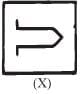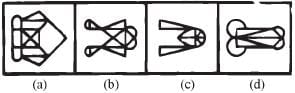Olympiad Test : Embedded Figure - 2

# Olympiad Test : Embedded Figure - 2

Test Description

## 10 Questions MCQ Test Maths Olympiad Class 6 | Olympiad Test : Embedded Figure - 2

Olympiad Test : Embedded Figure - 2 for Class 6 2023 is part of Maths Olympiad Class 6 preparation. The Olympiad Test : Embedded Figure - 2 questions and answers have been prepared according to the Class 6 exam syllabus.The Olympiad Test : Embedded Figure - 2 MCQs are made for Class 6 2023 Exam. Find important definitions, questions, notes, meanings, examples, exercises, MCQs and online tests for Olympiad Test : Embedded Figure - 2 below.
 1 Crore+ students have signed up on EduRev. Have you?
Olympiad Test : Embedded Figure - 2 - Question 1

### Analyze the set of figures and choose the correct option that contains figure X.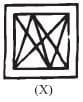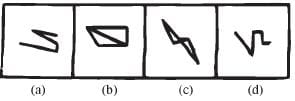Olympiad Test : Embedded Figure - 2 - Question 2

### Analyze the set of figures and choose the correct option that contains figure X.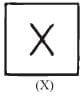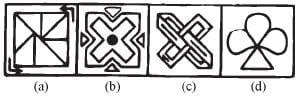Olympiad Test : Embedded Figure - 2 - Question 3

### Analyze the set of figures and choose the correct option that contains figure X.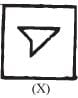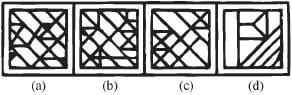Olympiad Test : Embedded Figure - 2 - Question 4

Analyze the set of figures and choose the correct option that contains figure X.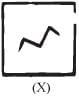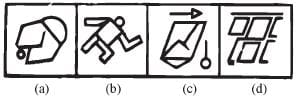Olympiad Test : Embedded Figure - 2 - Question 5

Analyze the set of figures and choose the correct option that contains figure X.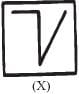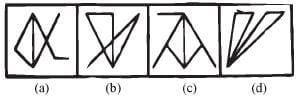Olympiad Test : Embedded Figure - 2 - Question 6

Analyze the set of figures and choose the correct option that contains figure X.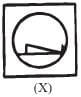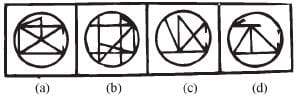Olympiad Test : Embedded Figure - 2 - Question 7

Analyze the set of figures and choose the correct option that contains figure X.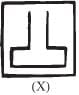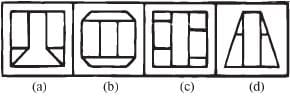Olympiad Test : Embedded Figure - 2 - Question 8

Analyze the set of figures and choose the correct option that contains figure X.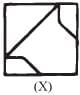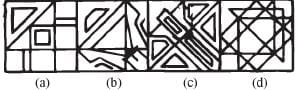Olympiad Test : Embedded Figure - 2 - Question 9

Analyze the set of figures and choose the correct option that contains figure X.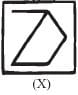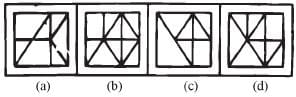Olympiad Test : Embedded Figure - 2 - Question 10

Analyze the set of figures and choose the correct option that contains figure X.